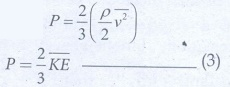Home | | Physics 11th std | Short Questions and Answer

## Chapter: 11th Physics : UNIT 9 : Kinetic Theory of Gases

Physics : Kinetic Theory of Gases : Book Back Important Questions, Answers, Solutions : Short Questions and Answer

Kinetic Theory of Gases (Physics)

1. What is the microscopic origin of pressure?

With the help of kinetic theory of gases, the pressure is linked to the velocity of molecules,V - Volume;- Avogadro velocity molecules,

m - mass of a molecule.

2. What is the microscopic origin of temperature?

Average Kinetic Energy / Molecule :

KE = ε = 3/2 NkT

3. Why moon has no atmosphere?

The escape speed of gases on the surfaces of Moon is much less than the root mean square speeds of gases due to low gravity. Due to this all the gases escape from the surface of the Moon.

4. Write the expression for rms speed, average speed and most probable speed of a gas molecule.5. What is the relation between the average kinetic energy and pressure?

The internal energy of the gas is given by

U = 3/2 NkT

The above equation can also be written as U = 3/2 PV, Since PV = NkT

P = 2/3 U/V = 2/3 u      …………(1)

From the equation (1), we can state that the pressure of the gas is equal to two thirds of internal energy per unit volume or internal energy density, u = U / V

Pressure in terms of mean kinetic energy density using equation.where ρ = nm = mass density (n is number density)

Multiply and divide R.H.S of equation (2) by 2, we getFrom the equation (3), pressure is equal to 2/3 of mean kinetic energy per unit volume.

6. Define the term degrees of freedom.

The minimum number of independent coordinates needed to specify the position and configuration of a thermo-dynamical system in space.

7. State the law of equipartition of energy.

According to kinetic theory, the average kinetic energy of system of molecules in thermal equilibrium at temperature T is uniformly distributed to all degrees of freedom will get ½ kT of energy. This is called law of equipartition of energy.

8. Define mean free path and write down its expression.

Average distance travelled by the molecule between collisions is called mean free path (λ). The mean free path based on kinetic theory.

9. Deduce Charles’ law based on kinetic theory.

Charles' law: From the equation

P = 2/3 (U/V ) = 2/3(u), we get PV = 2/3 (U)

For a fixed pressure, the volume of the gas is proportional to internal energy of the gas or average kinetic energy of the gas and the average kinetic energy is directly proportional to absolute temperature. It implies that V T or V / T = constant

10. Deduce Boyle’s law based on kinetic theory.

Boyle's law: From equation P = 2/3 (U/V) = 2/3 (u),

we know that PV = 2/3 U .

But the internal energy of an ideal gas is equal to N times the average kinetic energy () of each molecule. U = N

For a fixed temperature, the average translational kinetic energy will remain constant. It implies that PV = 2/3 N . Thus PV = constant

Therefore, pressure of a given gas is inversely proportional to its volume provided the temperature remains constant. This is Boyle's law.

11. Deduce Avogadro’s law based on kinetic theory.

This law states that at constant temperature and pressure, equal volumes of all gases contain the same number of molecules. For two different gases at the same temperature and pressure, according to kinetic theory of gases,

From equationare the mean square speed for two gases and N1 and N2 are the number of gas molecules in two different gases. At the same temperature, average kinetic energy per molecule is the same for two gases.………….(2)

Dividing the equation 1 by 2 we get N1 = N2

12. List the factors affecting the mean free path.

● Mean free path increases with increasing temperature. As the temperature increases, the average speed of each molecule will increase. It is the reason why the smell of hot sizzling food reaches several meter away than smell of cold food.

● Mean free path increases with decreasing pressure of the gas and diameter of the gas molecules.

13. What is the reason for Brownian motion?

According to kinetic theory, any particle suspended in a liquid or gas is continuously bombarded from all the directions so that the mean free path is almost negligible. This leads to the motion of the particles in a random and zig-zag manner.

Tags : Kinetic Theory of Gases | Physics , 11th Physics : UNIT 9 : Kinetic Theory of Gases
Study Material, Lecturing Notes, Assignment, Reference, Wiki description explanation, brief detail
11th Physics : UNIT 9 : Kinetic Theory of Gases : Short Questions and Answer | Kinetic Theory of Gases | Physics